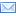#Difference Between Similar Terms and Objects

# Difference Between Mm and CmMm vs Cm

The metric system is an international system of measurement which is used by most countries as their basic system of measurement. It was designed for use by all, but it was found to be very useful in the fields of engineering, physics, astronomy, and other sciences.

It features interrelated base units which are equipped with prefixes to derive either a larger or a smaller unit. Prefixes multiply or divide measure by a factor of 10, 100, or 1,000 to come up with a smaller or larger unit of measure.
The meter is a base unit for the measurement of length adapted by the International System of Units (SI). Smaller units like the centimeter and the millimeter are derived from this basic unit by adding the prefixes “centi” which means 100, and “milli” which means 1,000.

Both the centimeter and the millimeter are part of the standard system of measurement that is used today by both scientists and the common person. These units of length are also determined by the International System of Units (SI).
The millimeter (mm) is a unit of length which is equal to one thousandth of a meter so a meter has 1,000 millimeters. It is also equivalent to 1,000 micrometers and 1,000,000 nanometers, and there are 25.4 mm in an inch. To illustrate how thick or thin a millimeter is, it is about as thick as a paper clip or a credit card. It is the measurement used to determine the amount of rainfall; 2 mm of rain would not be as disturbing as 15 mm of it which could already drench a person.

The centimeter (cm) is also a unit of length which is ten times larger than a millimeter and is equal to one hundredth of a meter; therefore, there are 100 centimeters in a meter. There are 2.54 centimeters in one inch. It is approximately the width of an adult fingernail, the width of a button, or of the keys in a piano. It is used as a practical unit of measurement in everyday measurements, and it is used in sewing to get the correct measurement of patterns. It is also the measurement by which snowfall is measured.

Summary:

1.A millimeter is a unit of length which is equal to one thousandth of a meter while a centimeter is a unit of length which is equal to one hundredth of a meter.
2.The millimeter is used to measure rainfall while the centimeter is used to measure snowfall.
3.While both have the meter as their base unit, the centimeter is ten times larger than a millimeter.
4.There are 25.4 millimeters in one inch while there are 2.54 centimeters in one inch.
5.The centimeter is used in everyday measurements rather than the millimeter.
6.The millimeter is about as thick as a credit card or a paper clip while a centimeter is about as thick as a button or an adult’s fingernail.

### Search DifferenceBetween.net :

Custom Search

Help us improve. Rate this post!Loading...Email This Post : If you like this article or our site. Please spread the word. Share it with your friends/family.

### 1 Comment

1. Thank You for the illustration of the paperclip and button, that
is the visual I needed.

Please note: comment moderation is enabled and may delay your comment. There is no need to resubmit your comment.

Articles on DifferenceBetween.net are general information, and are not intended to substitute for professional advice. The information is "AS IS", "WITH ALL FAULTS". User assumes all risk of use, damage, or injury. You agree that we have no liability for any damages.